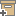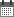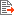Syntax
Search...
Manage
Community:
אסיף מאגר המחקר החקלאי
The functional dependence of canopy conductance on water vapor pressure deficit revisitedBack to searchPrevious item
Next item
Year:
2018
Authors :
Fuchs, Marcel
;
.
Volume :
Co-Authors:

Stanghellini C.

Facilitators :
From page:
1
To page:
10
(
Total pages:
10
)
Abstract:

Current research seeking to relate between ambient water vapor deficit (D) and foliage conductance (g F ) derives a canopy conductance (g W ) from measured transpiration by inverting the coupled transpiration model to yield g W = m - n ln(D) where m and n are fitting parameters. In contrast, this paper demonstrates that the relation between coupled g W and D is g W = AP/D + B, where P is the barometric pressure, A is the radiative term, and B is the convective term coefficient of the Penman-Monteith equation. A and B are functions of g F and of meteorological parameters but are mathematically independent of D. Keeping A and B constant implies constancy of g F . With these premises, the derived g W is a hyperbolic function of D resembling the logarithmic expression, in contradiction with the pre-set constancy of g F . Calculations with random inputs that ensure independence between g F and D reproduce published experimental scatter plots that display a dependence between g W and D in contradiction with the premises. For this reason, the dependence of g W on D is a computational artifact unrelated to any real effect of ambient humidity on stomatal aperture and closure. Data collected in a maize field confirm the inadequacy of the logarithmic function to quantify the relation between canopy conductance and vapor pressure deficit.

Note:
Related Files :
Air humidity
Coupling-interaction parameters
stomata
transpiration
Related Content
More details
DOI :
https://doi.org/10.1007/s0048
Article number:
0
Affiliations:
Database:
PubMed
Publication Type:
article
;
.
Language:
English
Editors' remarks:
ID:
36394
Last updated date:
02/03/2022 17:27
Creation date:
05/08/2018 12:19Scientific Publication
The functional dependence of canopy conductance on water vapor pressure deficit revisited

Stanghellini C.

The functional dependence of canopy conductance on water vapor pressure deficit revisited .

Current research seeking to relate between ambient water vapor deficit (D) and foliage conductance (g F ) derives a canopy conductance (g W ) from measured transpiration by inverting the coupled transpiration model to yield g W = m - n ln(D) where m and n are fitting parameters. In contrast, this paper demonstrates that the relation between coupled g W and D is g W = AP/D + B, where P is the barometric pressure, A is the radiative term, and B is the convective term coefficient of the Penman-Monteith equation. A and B are functions of g F and of meteorological parameters but are mathematically independent of D. Keeping A and B constant implies constancy of g F . With these premises, the derived g W is a hyperbolic function of D resembling the logarithmic expression, in contradiction with the pre-set constancy of g F . Calculations with random inputs that ensure independence between g F and D reproduce published experimental scatter plots that display a dependence between g W and D in contradiction with the premises. For this reason, the dependence of g W on D is a computational artifact unrelated to any real effect of ambient humidity on stomatal aperture and closure. Data collected in a maize field confirm the inadequacy of the logarithmic function to quantify the relation between canopy conductance and vapor pressure deficit.

Scientific Publication
You may also be interested in